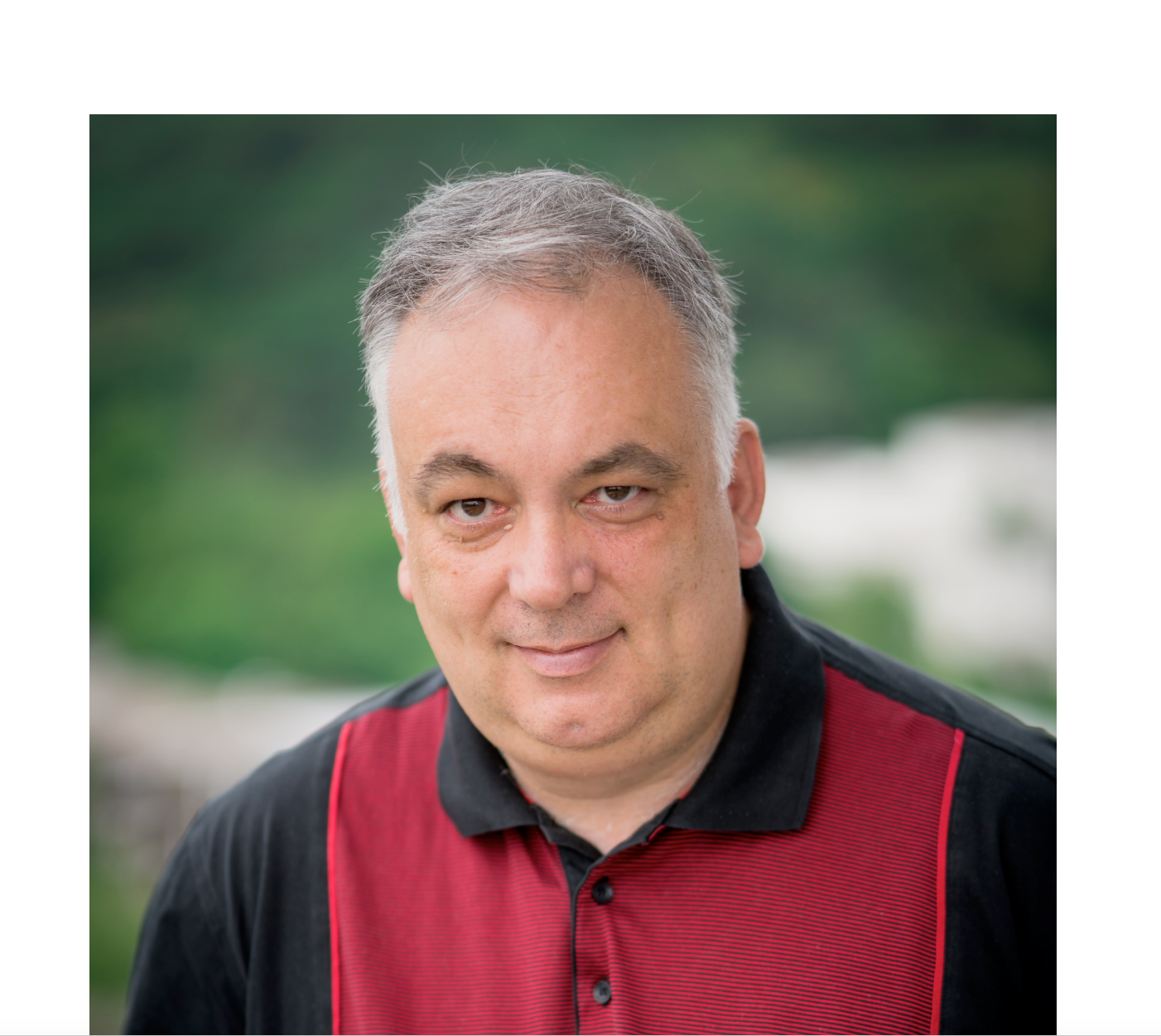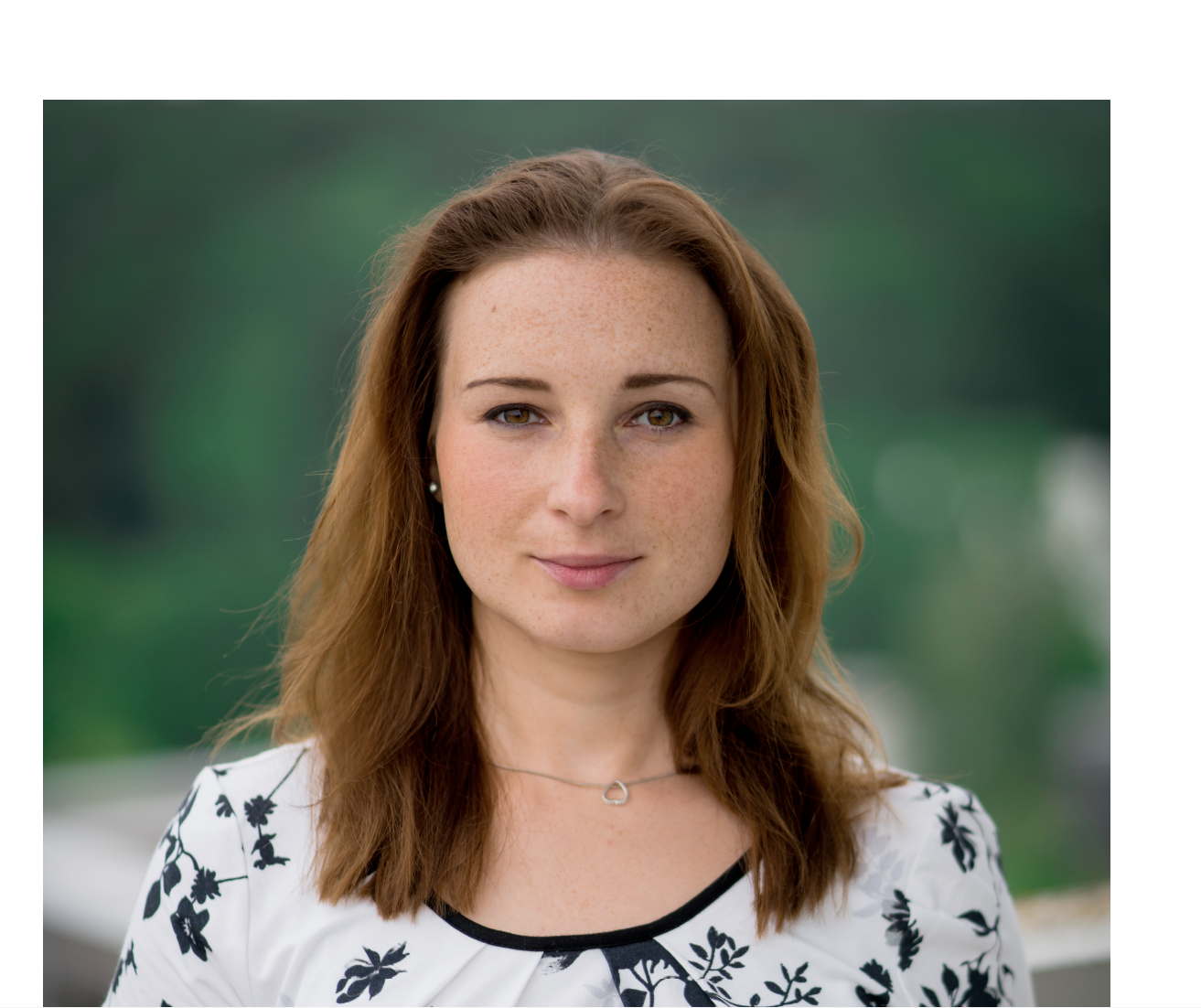# Timo Weidl

Prof. Dr.

Professor - Lehrstuhl für Analysis und Mathematische Physik
Institut für Analysis, Dynamik und Modellierung
Lehrstuhl für Analysis und Mathematische Physik
[Foto: Robin Lang, 2018]

## Kontakt

+4971168565594

Website
Visitenkarte (VCF)

Pfaffenwaldring 57
70569 Stuttgart
Deutschland
Raum: 8.347

## Sprechstunde

nach Vereinbarung

## Fachgebiet

Der Schwerpunkt meiner Forschung liegt in der Spektralanalyse von Differential- und Integraloperatoren mit Anwendungen in der Quantenmechanik.

1. R. Frank, A. Laptev, und T. Weidl, Schrödinger Operators: Eigenvalues and Lieb–Thirring Inequalities. Cambridge University Press, 2022, S. 512.
2. R. L. Frank, A. Laptev, und T. Weidl, „An improved one-dimensional Hardy inequality“. https://arxiv.org/abs/2204.00877, S. 19, 2022. [Online]. Verfügbar unter: https://arxiv.org/abs/2204.00877
3. R. L. Frank, A. Laptev, und T. Weidl, „An improved one-dimensional Hardy inequality“, J. Math. Sci. (N.Y.), Bd. 268, Nr. 3, Problems in mathematical analysis. No. 118, Art. Nr. 3, Problems in mathematical analysis. No. 118, 2022, doi: 10.1007/s10958-022-06199-8.
4. R. D. Benguria u. a., Partial differential equations, spectral theory, and mathematical physics—the Ari Laptev anniversary volume. in EMS Series of Congress Reports. EMS Press, Berlin, 2021. doi: 10.4171/ECR/18.
5. H. Kovar\’ık, B. Ruszkowski, und T. Weidl, „Melas-type bounds for the Heisenberg Laplacian on bounded domains“, Journal of Spectral Theory, Bd. 8, Nr. 2, Art. Nr. 2, Feb. 2018, doi: 10.4171/jst/200.
6. A. Hänel und T. Weidl, „Spectral asymptotics for the Dirichlet Laplacian with a Neumann window via a Birman-Schwinger analysis of the Dirichlet-to-Neumann operator.“, Functional Analysis and Operator Theory for Quantum Physics, EMS Series of Congress Reports, J. Dittrich, et al. (eds.), S. 315–352, 2017.
7. H. Kovarik, B. Ruszkowski, und T. Weidl, „Spectral estimates for the Heisenberg Laplacian on cylinders.“, Functional Analysis and Operator Theory for Quantum Physics, EMS Series of Congress Reports, J. Dittrich, et al. (eds.), S. 433–446, 2017.
8. A. Hänel und T. Weidl, „Eigenvalue asymptotics for an elastic strip and an elastic plate with a crack.“, Quarterly journal of mechanics and applied mathematics, Bd. 69, Nr. 4, Art. Nr. 4, 2016, doi: 10.1093/qjmam/hbw009.
9. D. Barseghyan, P. Exner, H. Kovarik, und T. Weidl, „Semiclassical bounds in magnetic bottles“, Reviews in Mathematical Physics, Bd. 28, Nr. 1, Art. Nr. 1, 2016, doi: 10.1142/S0129055X16500021.
10. H. Kovar\’ık und T. Weidl, „Improved Berezin-Li-Yau inequalities with magnetic field“, Proc. Roy. Soc. Edinburgh Sect. A, Bd. 145, Nr. 1, Art. Nr. 1, 2015, doi: 10.1017/S0308210513001595.
11. C. Förster und T. Weidl, „Trapped modes in an elastic plate with a hole.“, St. Petersburg Mathematical Journal, Bd. 23, Nr. 1, Art. Nr. 1, 2012.
12. L. Geisinger, A. Laptev, und T. Weidl, „Geometrical versions of improved Berezin-Li-Yau inequalities.“, Journal of Spectral Theory 1., Nr. 1, Art. Nr. 1, 2011, [Online]. Verfügbar unter: https://arxiv.org/pdf/1010.2683.pdf
13. T. Weidl, „Semiclassical Spectral Bounds and Beyond.“, Mathematical results in quantum physics, S. 110–129, 2011, doi: 10.1142/9789814350365_0009.
14. L. Geisinger und T. Weidl, „Sharp spectral estimates in domains of infinite volume.“, Reviews in Mathematical Physics., Bd. 23, Nr. 6, Art. Nr. 6, 2011, doi: 10.1142/S0129055X11004394.
15. C. Förster und T. Weidl, „Trapped modes in an elastic plate with a hole.“, Rossiĭskaya Akademiya Nauk. Algebra i Analiz, Bd. 23, Nr. 1, Art. Nr. 1, 2011.
16. L. Geisinger und T. Weidl, „Universal bounds for traces of the Dirichlet Laplace operator.“, Journal of the London Mathematical Society. Second Series, Bd. 82, Nr. 2, Art. Nr. 2, 2010, doi: https://doi.org/10.1112/jlms/jdq033.
17. H. Kovar\’ık, S. Vugalter, und T. Weidl, „Two-dimensional Berezin-Li-Yau inequalities with a correction term“, Comm. Math. Phys., Bd. 287, Nr. 3, Art. Nr. 3, 2009, doi: 10.1007/s00220-008-0692-1.
18. R. L. Frank, M. Loss, und T. Weidl, „Pólya’s conjecture in the presence of a constant magnetic field“, J. Eur. Math. Soc. (JEMS), Bd. 11, Nr. 6, Art. Nr. 6, 2009, doi: 10.4171/JEMS/184.
19. T. Weidl, „Improved Berezin-Li-Yau inequalities with a remainder term“, in Spectral theory of differential operators, in Spectral theory of differential operators, vol. 225. Amer. Math. Soc., Providence, RI, 2008, S. 253--263. doi: 10.1090/trans2/225/17.
20. R. L. Frank, B. Simon, und T. Weidl, „Eigenvalue bounds for perturbations of Schrödinger operators and Jacobi matrices with regular ground states“, Comm. Math. Phys., Bd. 282, Nr. 1, Art. Nr. 1, 2008, doi: 10.1007/s00220-008-0453-1.
21. T. Weidl, „Nonstandard Cwikel type estimates“, in Interpolation theory and applications, in Interpolation theory and applications, vol. 445. Amer. Math. Soc., Providence, RI, 2007, S. 337--357. doi: 10.1090/conm/445/08611.
22. H. Kovar\’ık, S. Vugalter, und T. Weidl, „Spectral estimates for two-dimensional Schrödinger operators with application to quantum layers“, Comm. Math. Phys., Bd. 275, Nr. 3, Art. Nr. 3, 2007, doi: 10.1007/s00220-007-0318-z.
23. C. Förster und T. Weidl, „Trapped modes for an elastic strip with perturbation of the material properties“, Quart. J. Mech. Appl. Math., Bd. 59, Nr. 3, Art. Nr. 3, 2006, doi: 10.1093/qjmam/hbl008.
24. P. Exner, H. Linde, und T. Weidl, „Lieb-Thirring inequalities for geometrically induced bound states“, Lett. Math. Phys., Bd. 70, Nr. 1, Art. Nr. 1, 2004, doi: 10.1007/s11005-004-1741-0.
25. S. Vugalter und T. Weidl, „On the discrete spectrum of a pseudo-relativistic two-body pair operator“, Ann. Henri Poincaré, Bd. 4, Nr. 2, Art. Nr. 2, 2003, doi: 10.1007/s00023-003-0131-y.
26. A. Laptev, O. Safronov, und T. Weidl, „Bound state asymptotics for elliptic operators with strongly degenerated symbols“, in Nonlinear problems in mathematical physics and related topics, I, in Nonlinear problems in mathematical physics and related topics, I, vol. 1. Kluwer/Plenum, New York, 2002, S. 233--246. doi: 10.1007/978-1-4615-0777-2\_14.
27. P. Exner und T. Weidl, „Lieb-Thirring inequalities on trapped modes in quantum wires“, in XIIIth International Congress on Mathematical Physics (London, 2000), in XIIIth International Congress on Mathematical Physics (London, 2000). Int. Press, Boston, MA, 2001, S. 437--443.
28. A. Laptev und T. Weidl, „Recent results on Lieb-Thirring inequalities“, in Journées Équations aux Dérivées Partielles’’ (La Chapelle sur Erdre, 2000), in Journées Équations aux Dérivées Partielles’’ (La Chapelle sur Erdre, 2000). Univ. Nantes, Nantes, 2000, S. Exp. No. XX, 14.
29. D. Hundertmark, A. Laptev, und T. Weidl, „New bounds on the Lieb-Thirring constants“, Invent. Math., Bd. 140, Nr. 3, Art. Nr. 3, 2000, doi: 10.1007/s002220000077.
30. A. Laptev und T. Weidl, „Sharp Lieb-Thirring inequalities in high dimensions“, Acta Math., Bd. 184, Nr. 1, Art. Nr. 1, 2000, doi: 10.1007/BF02392782.
31. T. Weidl, „Remarks on virtual bound states for semi-bounded operators“, Comm. Partial Differential Equations, Bd. 24, Nr. 1–2, Art. Nr. 1–2, 1999, doi: 10.1080/03605309908821417.
32. T. Weidl, „A remark on Hardy type inequalities for critical Schrödinger operators with magnetic fields“, in The Mazya anniversary collection, Vol. 2 (Rostock,              1998), in The Mazya anniversary collection, Vol. 2 (Rostock,              1998), vol. 110. Birkhäuser, Basel, 1999, S. 345--352.
33. A. Laptev und T. Weidl, „Hardy inequalities for magnetic Dirichlet forms“, in Mathematical results in quantum mechanics (Prague, 1998), in Mathematical results in quantum mechanics (Prague, 1998), vol. 108. Birkhäuser, Basel, 1999, S. 299--305.
34. T. Weidl, „Another look at Cwikel’s inequality“, in Differential operators and spectral theory, in Differential operators and spectral theory, vol. 189. Amer. Math. Soc., Providence, RI, 1999, S. 247--254. doi: 10.1090/trans2/189/19.
35. T. Weidl, „Eigenvalue asymptotics for locally perturbed second-order differential operators“, J. London Math. Soc. (2), Bd. 59, Nr. 1, Art. Nr. 1, 1999, doi: 10.1112/S0024610799007024.
36. I. Roitberg, D. Vassiliev, und T. Weidl, „Edge resonance in an elastic semi-strip“, Quart. J. Mech. Appl. Math., Bd. 51, Nr. 1, Art. Nr. 1, 1998, doi: 10.1093/qjmam/51.1.1.
37. Y. Netrusov und T. Weidl, „On Lieb-Thirring inequalities for higher order operators with critical and subcritical powers“, Comm. Math. Phys., Bd. 182, Nr. 2, Art. Nr. 2, 1996, [Online]. Verfügbar unter: http://projecteuclid.org/euclid.cmp/1104288152
38. T. Weidl, „On the Lieb-Thirring constants $L_\gamma,1$ for $\gamma1/2$“, Comm. Math. Phys., Bd. 178, Nr. 1, Art. Nr. 1, 1996, [Online]. Verfügbar unter: http://projecteuclid.org/euclid.cmp/1104286558
39. T. Weidl, On the discrete spectrum of partial differential and integral operators. ProQuest LLC, Ann Arbor, MI, 1995, S. 136. [Online]. Verfügbar unter: http://gateway.proquest.com/openurl?url_ver=Z39.88-2004&rft_val_fmt=info:ofi/fmt:kev:mtx:dissertation&res_dat=xri:pqdiss&rft_dat=xri:pqdiss:C522556
40. T. Weidl, „Cwikel type estimates in non-power ideals“, Math. Nachr., Bd. 176, S. 315--334, 1995, doi: 10.1002/mana.19951760123.
41. T. Weidl, „Estimates for operators of the form b(x)a(D)  in non-powerlike ideals.“, St.-Petersburg Mathematical Journal, Bd. 5, Nr. 5, Art. Nr. 5, 1994.
42. M. Sh. Birman und T. Weidl, „The discrete spectrum in a gap of the continuous one for compact supported perturbations“, in Mathematical results in quantum mechanics (Blossin, 1993), in Mathematical results in quantum mechanics (Blossin, 1993), vol. 70. Birkhäuser, Basel, 1994, S. 9--12. doi: 10.1007/978-3-0348-8545-4\_2.
43. T. Vaıdl\cprime, „Estimates for operators of type $b(x)a(D)$ in nonpower ideals“, Algebra i Analiz, Bd. 5, Nr. 5, Art. Nr. 5, 1993.
44. T. Vaıdl\cprime, „General operator ideals of weak type“, Algebra i Analiz, Bd. 4, Nr. 3, Art. Nr. 3, 1992.

### Kontakt:### Timo Weidl

Prof. Dr.

Professor - Lehrstuhl für Analysis und Mathematische Physik

[Foto: Robin Lang, 2018]### Elke Peter

Sekretariat am Lehrstuhl für Analysis und Mathematische Physik

[Foto: Robin Lang, 2018]